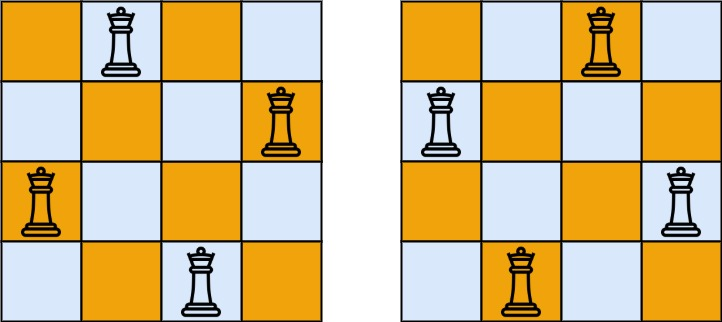# GeetCode Hub

The n-queens puzzle is the problem of placing `n` queens on an `n x n` chessboard such that no two queens attack each other.

Given an integer `n`, return the number of distinct solutions to the n-queens puzzle.

Example 1:```Input: n = 4
Output: 2
Explanation: There are two distinct solutions to the 4-queens puzzle as shown.
```

Example 2:

```Input: n = 1
Output: 1
```

Constraints:

• `1 <= n <= 9`

class Solution { public int totalNQueens(int n) { } }